# Category: games

Last time, we’ve viewed major and minor triads (chords) as inscribed triangles in a regular $12$-gon.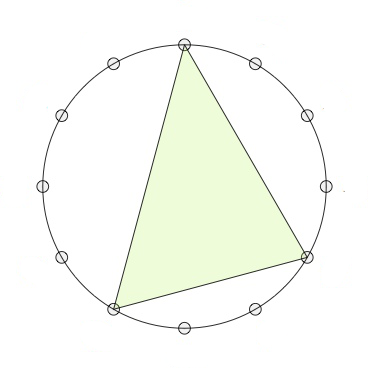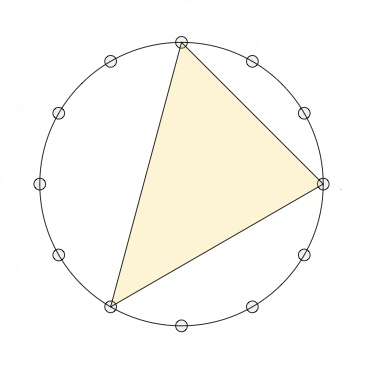If we move clockwise along the $12$-gon, starting from the endpoint of the longest edge (the root of the chord, here the $0$-vertex) the edges skip $3,2$ and $4$ vertices (for a major chord, here on the left the major $0$-chord) or $2,3$ and $4$ vertices (for a minor chord, here on the right the minor $0$-chord).

The symmetries of the $12$-gon, the dihedral group $D_{12}$, act on the $24$ major- and minor-chords transitively, preserving the type for rotations, and interchanging majors with minors for reflections.

Mathematical Music Theoreticians (MaMuTh-ers for short) call this the $T/I$-group, and view the rotations of the $12$-gon as transpositions $T_k : x \mapsto x+k~\text{mod}~12$, and the reflections as involutions $I_k : x \mapsto -x+k~\text{mod}~12$.

Note that the elements of the $T/I$-group act on the vertices of the $12$-gon, from which the action on the chord-triangles follows.

There is another action on the $24$ major and minor chords, mapping a chord-triangle to its image under a reflection in one of its three sides.

Note that in this case the reflection $I_k$ used will depend on the root of the chord, so this action on the chords does not come from an action on the vertices of the $12$-gon.

There are three such operations: (pictures are taken from Alexandre Popoff’s blog, with the ‘funny names’ removed)

The $P$-operation is reflection in the longest side of the chord-triangle. As the longest side is preserved, $P$ interchanges the major and minor chord with the same root.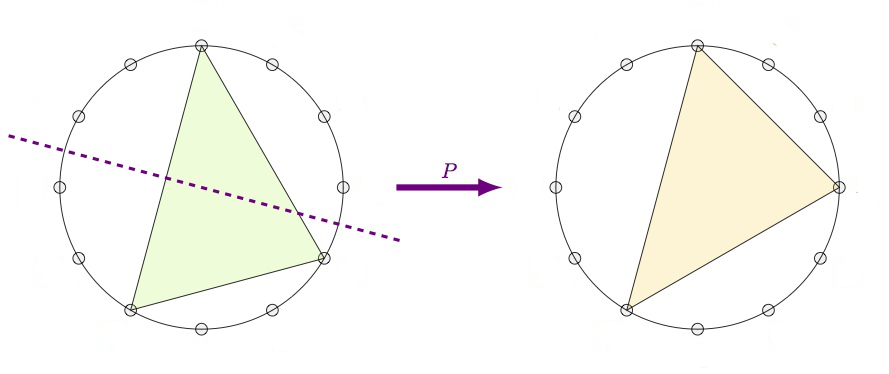The $L$-operation is refection in the shortest side. This operation interchanges a major $k$-chord with a minor $k+4~\text{mod}~12$-chord.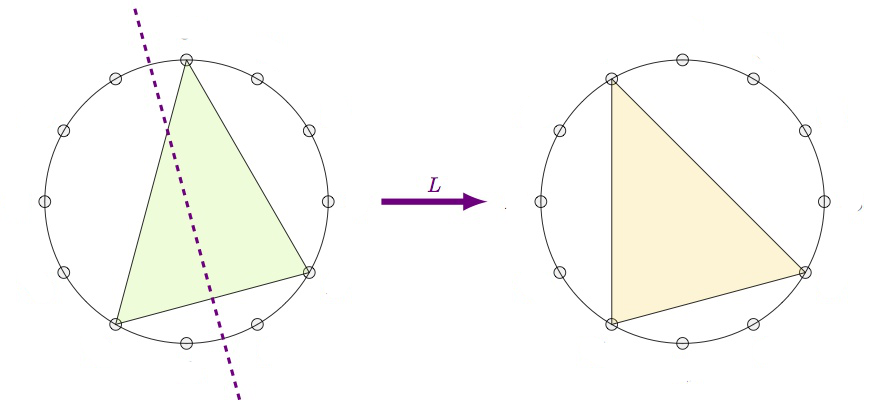Finally, the $R$-operation is reflection in the middle side. This operation interchanges a major $k$-chord with a minor $k+9~\text{mod}~12$-chord.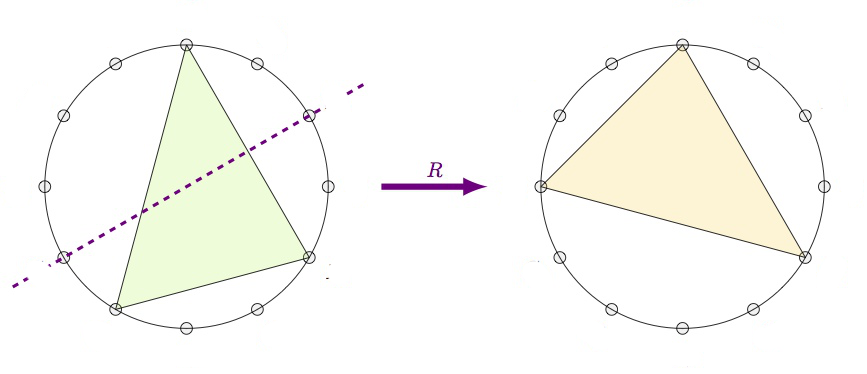From this it is already clear that the group generated by $P$, $L$ and $R$ acts transitively on the $24$ major and minor chords, but what is this $PLR$-group?

If we label the major chords by their root-vertex $1,2,\dots,12$ (GAP doesn’t like zeroes), and the corresponding minor chords $13,14,\dots,24$, then these operations give these permutations on the $24$ chords:

 P:=(1,13)(2,14)(3,15)(4,16)(5,17)(6,18)(7,19)(8,20)(9,21)(10,22)(11,23)(12,24) L:=(1,17)(2,18)(3,19)(4,20)(5,21)(6,22)(7,23)(8,24)(9,13)(10,14)(11,15)(12,16) R:=(1,22)(2,23)(3,24)(4,13)(5,14)(6,15)(7,16)(8,17)(9,18)(10,19)(11,20)(12,21) 

Then GAP gives us that the $PLR$-group is again isomorphic to $D_{12}$:

 gap> G:=Group(P,L,R);; gap> Size(G); 24 gap> IsDihedralGroup(G); true 

In fact, if we view both the $T/I$-group and the $PLR$-group as subgroups of the symmetric group $Sym(24)$ via their actions on the $24$ major and minor chords, these groups are each other centralizers! That is, the $T/I$-group and $PLR$-group are dual to each other.

For more on this, there’s a beautiful paper by Alissa Crans, Thomas Fiore and Ramon Satyendra: Musical Actions of Dihedral Groups.

What does this new MaMuTh info learns us more about our Elephant, the Topos of Triads, studied by Thomas Noll?

Last time we’ve seen the eight element triadic monoid $T$ of all affine maps preserving the three tones $\{ 0,4,7 \}$ of the major $0$-chord, computed the subobject classified $\Omega$ of the corresponding topos of presheaves, and determined all its six Grothendieck topologies, among which were these three: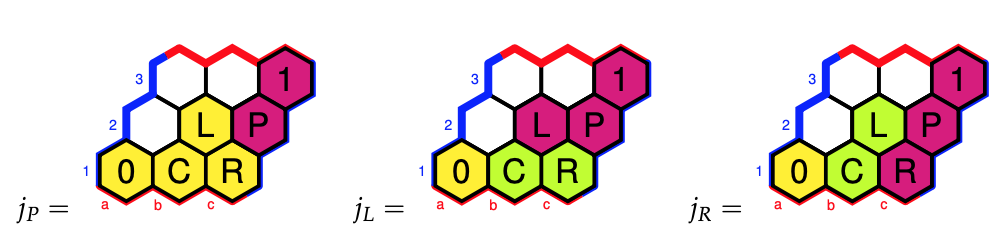Why did we label these Grothendieck topologies (and corresponding elements of $\Omega$) by $P$, $L$ and $R$?

We’ve seen that the sheafification of the presheaf $\{ 0,4,7 \}$ in the triadic topos under the Grothendieck topology $j_P$ gave us the sheaf $\{ 0,3,4,7 \}$, and these are the tones of the major $0$-chord together with those of the minor $0$-chord, that is the two chords in the $\langle P \rangle$-orbit of the major $0$-chord. The group $\langle P \rangle$ is the cyclic group $C_2$.

For the sheafication with respect to $j_L$ we found the $T$-set $\{ 0,3,4,7,8,11 \}$ which are the tones of the major and minor $0$-,$4$-, and $8$-chords. Again, these are exactly the six chords in the $\langle P,L \rangle$-orbit of the major $0$-chord. The group $\langle P,L \rangle$ is isomorphic to $Sym(3)$.

The $j_R$-topology gave us the $T$-set $\{ 0,1,3,4,6,7,9,10 \}$ which are the tones of the major and minor $0$-,$3$-, $6$-, and $9$-chords, and lo and behold, these are the eight chords in the $\langle P,R \rangle$-orbit of the major $0$-chord. The group $\langle P,R \rangle$ is the dihedral group $D_4$.

More on this can be found in the paper Commuting Groups and the Topos of Triads by Thomas Fiore and Thomas Noll.

The operations $P$, $L$ and $R$ on major and minor chords are reflexions in one side of the chord-triangle, so they preserve two of the three tones. There’s a distinction between the $P$ and $L$ operations and $R$ when it comes to how the third tone changes.

Under $P$ and $L$ the third tone changes by one halftone (because the corresponding sides skip an even number of vertices), whereas under $R$ the third tone changes by two halftones (a full tone), see the pictures above.

The $\langle P,L \rangle = Sym(3)$ subgroup divides the $24$ chords in four orbits of six chords each, three major chords and their corresponding minor chords. These orbits consist of the

• $0$-, $4$-, and $8$-chords (see before)
• $1$-, $5$-, and $9$-chords
• $2$-, $6$-, and $10$-chords
• $3$-, $7$-, and $11$-chords

and we can view each of these orbits as a cycle tracing six of the eight vertices of a cube with one pair of antipodal points removed.

These four ‘almost’ cubes are the NE-, SE-, SW-, and NW-regions of the Cube Dance Graph, from the paper Parsimonious Graphs by Jack Douthett and Peter Steinbach.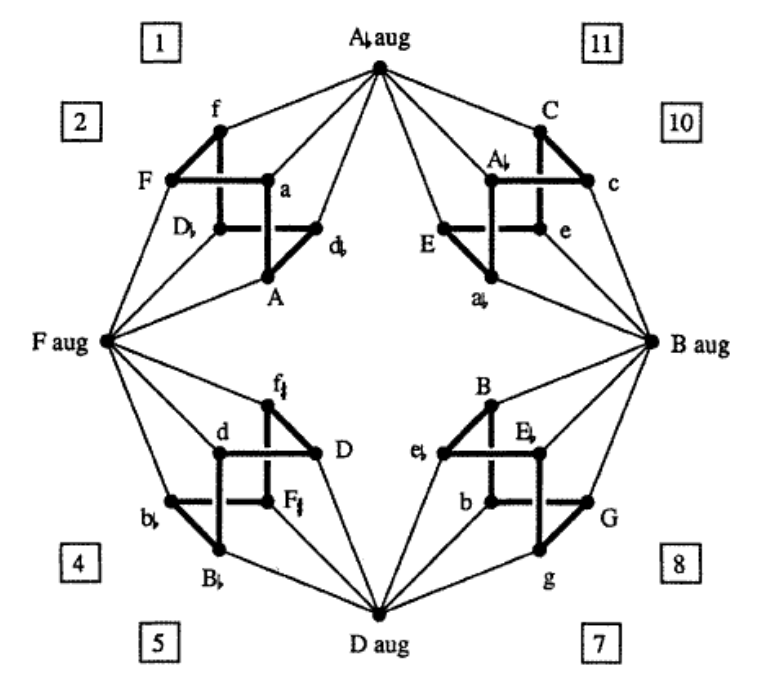To translate the funny names to our numbers, use this dictionary (major chords are given by a capital letter):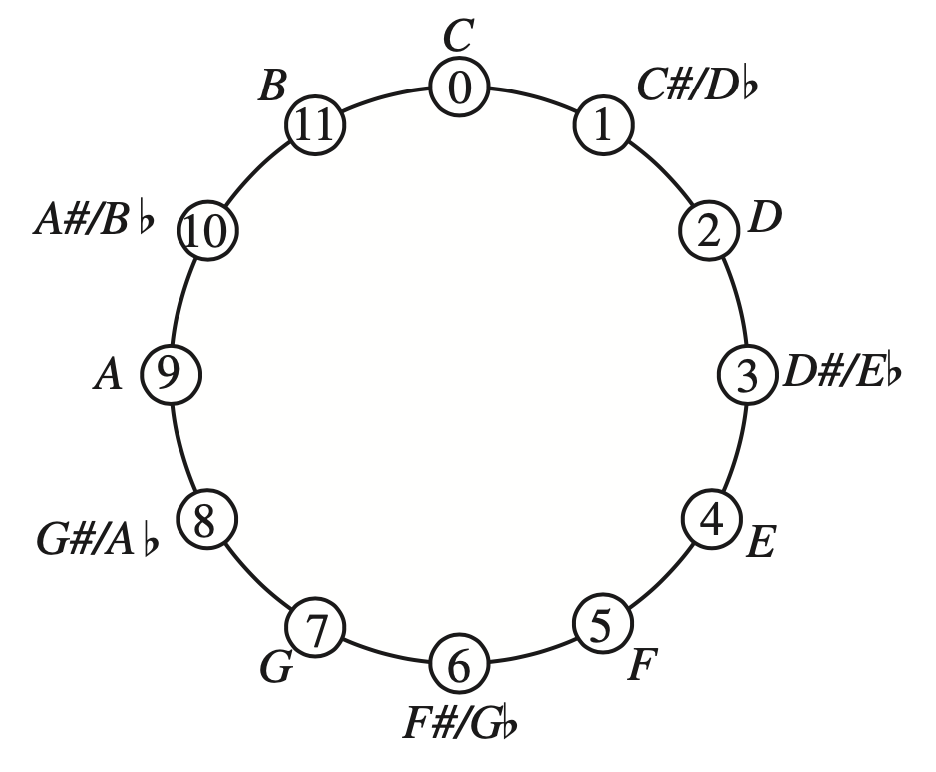The four extra chords (at the N, E, S, and P places) are augmented triads. They correspond to the triads $(0,4,8),~(1,5,9),~(2,6,10)$ and $(3,7,11)$.

That is, two triads are connected by an edge in the Cube Dance graph if they share two tones and differ by an halftone in the third tone.

This graph screams for a group or monoid acting on it. Some of the edges we’ve already identified as the action of $P$ and $L$ on the $24$ major and minor triads. Because the triangle of an augmented triad is equilateral, we see that they are preserved under $P$ and $L$.

But what about the edges connecting the regular triads to the augmented ones? If we view each edge as two directed arrows assigned to the same operation, we cannot do this with a transformation because the operation sends each augmented triad to six regular triads.

Alexandre Popoff, Moreno Andreatta and Andree Ehresmann suggest in their paper Relational poly-Klumpenhouwer networks for transformational and voice-leading analysis that one might use a monoid generated by relations, and they show that there is such a monoid with $40$ elements acting on the Cube Dance graph.

Popoff claims that usual presheaf toposes, that is contravariant functors to $\mathbf{Sets}$ are not enough to study transformational music theory. He suggest to use instead functors to $\mathbf{Rel}$, that is Sets with as the morphisms binary relations, and their compositions.

Another Elephant enters the room…

(to be continued)

Here, MaMuTh stands for Mathematical Music Theory which analyses the pitch, timing, and structure of works of music.

The Elephant is the nickname for the ‘bible’ of topos theory, Sketches of an Elephant: A Topos Theory Compendium, a two (three?) volume book, written by Peter Johnstone.

How can we get as quickly as possible from the MaMuth to the Elephant, musical illiterates such as myself?

What Mamuth-ers call a pitch class (sounds that are a whole number of octaves apart), is for us a residue modulo $12$, as an octave is usually divided into twelve (half)tones.

We’ll just denote them by numbers from $0$ to $11$, or view them as the vertices of a regular $12$-gon, and forget the funny names given to them, as there are several such encodings, and we don’t know a $G$ from a $D\#$.Our regular $12$-gon has exactly $24$ symmetries. Twelve rotations, which they call transpositions, given by the affine transformations
$T_k~:~x \mapsto x+k~\text{mod}~12$
and twelve reflexions, which they call involutions, given by
$I_k~:~x \mapsto -x+k~\text{mod}~12$
What for us is the dihedral group $D_{12}$ (all symmetries of the $12$-gon), is for them the $T/I$-group (for transpositions/involutions).

Let’s move from individual notes (or pitch classes) to chords (or triads), that is, three notes played together.

Not all triples of notes sound nice when played together, that’s why the most commonly played chords are among the major and minor triads.

A major triad is an ordered triple of elements from $\mathbb{Z}_{12}$ of the form
$(n,n+4~\text{mod}~12,n+7~\text{mod}~12)$
and a minor triad is an ordered triple of the form
$(n,n+3~\text{mod}~12,n+7~\text{mod}~12)$
where the first entry $n$ is called the root of the triad (or chord) and its funny name is then also the name of that chord.

For us, it is best to view a triad as an inscribed triangle in our regular $12$-gon. The triangles of major and minor triads have edges of different lengths, a small one, a middle, and a large one.

Starting from the root, and moving clockwise, we encounter in a major chord-triangle first the middle edge, then the small edge, and finally the large edge. For a minor chord-triangle, we have first the small edge, then the middle one, and finally the large edge.

On the left, two major triads, one with root $0$, the other with root $6$. On the right, two minor triads, also with roots $0$ and $6$.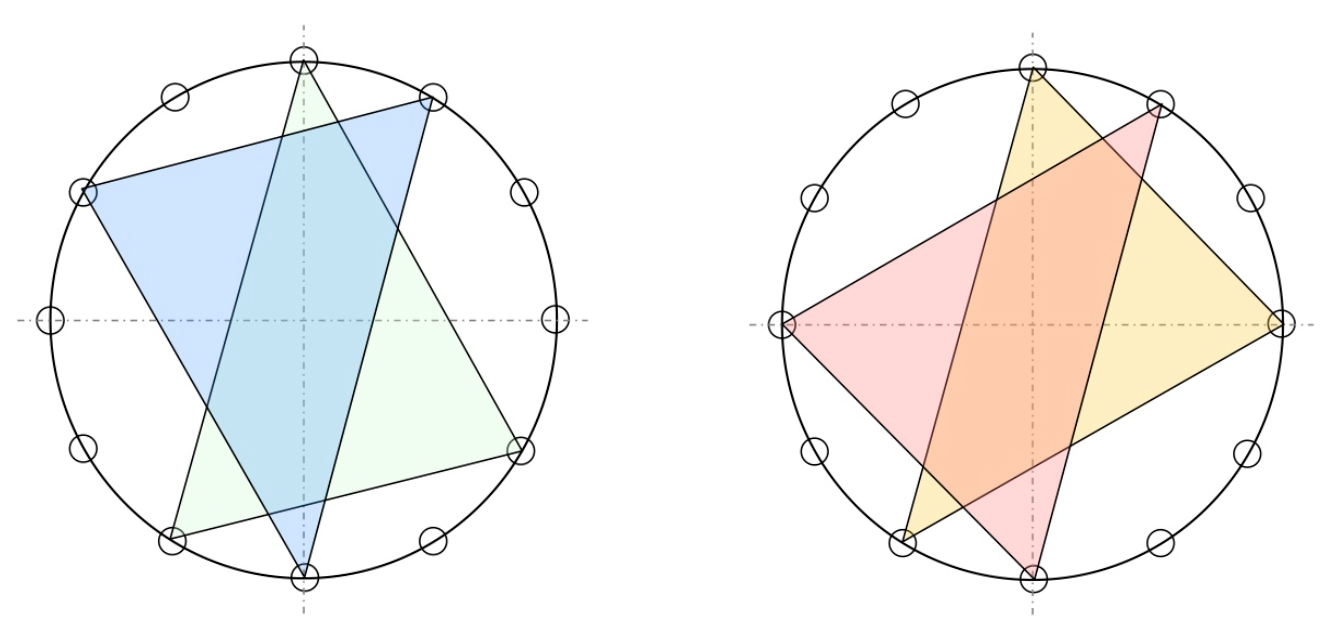(Btw. if you are interested in the full musical story, I strongly recommend the alpof blog by Alexandre Popoff, from which the above picture is taken.)

Clearly, there are $12$ major triads (one for each root), and $12$ minor triads.

From the shape of the triad-triangles it is also clear that rotations (transpositions) send major triads to major triads (and minors to minors), and that reflexions (involutions) interchange major with minor triads.

That is, the dihedral group $D_{12}$ (or if you prefer the $T/I$-group) acts on the set of $24$ major and minor triads, and this action is transitive (an element stabilising a triad-triangle must preserve its type (so is a rotation) and its root (so must be the identity)).

Can we hear the action of the very special group element $T_6$ (the unique non-trivial central element of $D_{12}$) on the chords?

This action is not only the transposition by three full tones, but also a point-reflexion with respect to the center of the $12$-gon (see two examples in the picture above). This point reflexion can be compositionally meaningful to refer to two very different upside-down worlds.

In It’s $T_6$-day, Alexandre Popoff gives several examples. Here’s one of them, the Ark theme in Indiana Jones – Raiders of the Lost Ark.

“The $T_6$ transformation is heard throughout the map room scene (in particular at 2:47 in the video): that the ark is a dreadful object from a very different world is well rendered by the $T_6$ transposition, with its inherent tritone and point reflection.”

Let’s move on in the direction of the Elephant.

We saw that the only affine map of the form $x \mapsto \pm x + k$ fixing say the major $0$-triad $(0,4,7)$ is the identity map.

But, we can ask for the collection of all affine maps $x \mapsto a x + b$ fixing this major $0$-triad set-wise, that is, such that
$\{ b, 4a+b~\text{mod}~12, 7a+b~\text{mod}~2 \} \subseteq \{ 0,4,7 \}$

A quick case-by-case analysis shows that there are just eight such maps: the identity and the constant maps
$x \mapsto x,~x \mapsto 0,~x \mapsto 4, ~x \mapsto 7$
and the four maps
$\underbrace{x \mapsto 3x+7}_a,~\underbrace{x \mapsto 8x+4}_b,~x \mapsto 9x+4,~x \mapsto 4x$

Compositions of such maps again preserve the set $\{ 0,4,7 \}$ so they form a monoid, and a quick inspection with GAP learns that $a$ and $b$ generate this monoid.

 gap> a:=Transformation([10,1,4,7,10,1,4,7,10,1,4,7]);; gap> b:=Transformation([12,8,4,12,8,4,12,8,4,12,8,4]);; gap> gens:=[a,b];; gap> T:=Monoid(gens); gap> Size(T); 8 

The monoid $T$ is the triadic monoid of Thomas Noll’s paper The topos of triads.

The monoid $T$ can be seen as a one-object category (with endomorphisms the elements of $T$). The corresponding presheaf topos is then the category of all sets equipped with a right $T$-action.

Actually, Noll considers just one such presheaf (and its sub-presheaves) namely $\mathcal{F}=\mathbb{Z}_{12}$ with the action of $T$ by affine maps described before.

He is interested in the sheafifications of these presheaves with respect to Grothendieck topologies, so we have to describe those.

For any monoid category, the subobject classifier $\Omega$ is the set of all right ideals in the monoid.

Using the GAP sgpviz package we can draw its Cayley graph (red coloured vertices are idempotents in the monoid, the blue vertex is the identity map).

 gap> DrawCayleyGraph(T);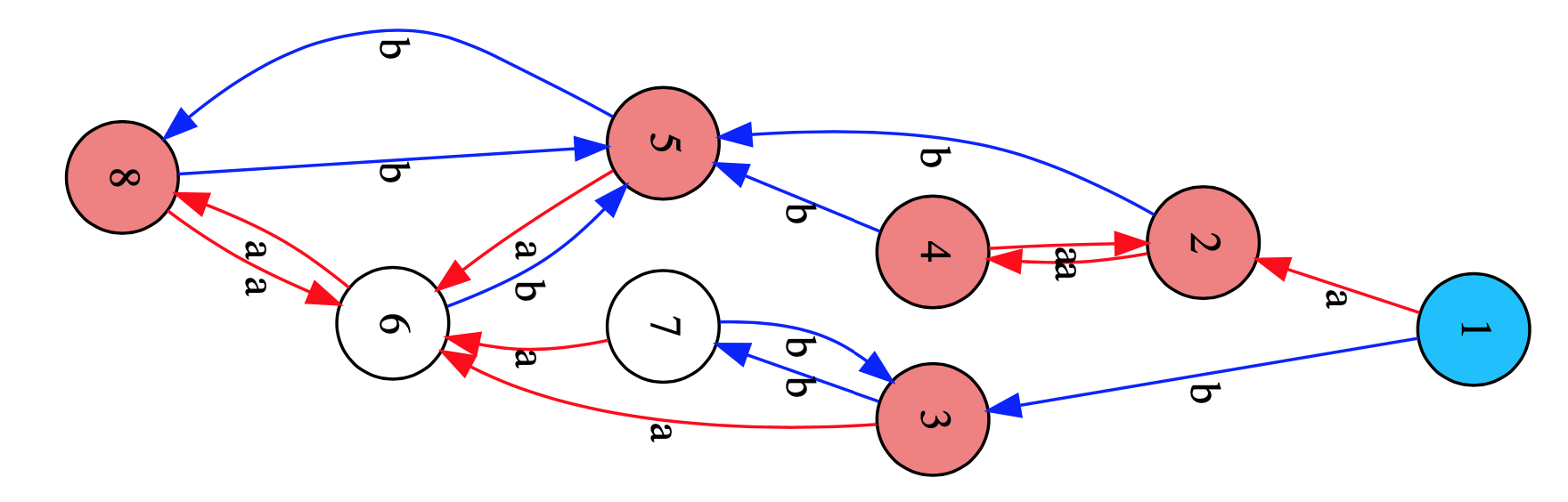The elements of $T$ (vertices) which can be connected by oriented paths (in both ways) in the Cayley graph, such as here $\{ 2,4 \}$, $\{ 3,7 \}$ and $\{ 5,6,8 \}$, will generate the same right ideal in $T$, so distinct right ideals are determined by unidirectional arrows, such as from $1$ to $2$ and $3$ or from $\{ 2,4 \}$ to $5$, or from $\{ 3,7 \}$ to $6$.

This gives us that $\Omega$ consists of the following six elements:

• $0 = \emptyset$
• $C = \{ 5,6,8 \} = a.T \wedge b.T$
• $L = \{ 2,4,5,6,8 \}=a.T$
• $R = \{ 3,7,5,6,8 \}=b.T$
• $P = \{ 2,3,4,5,6,7,8 \}=a.T \vee b.T$
• $1 = T$

As a subobject classifier $\Omega$ is itself a presheaf, so wat is the action of the triad monoid $T$ on it? For all $A \in \Omega$, and $s \in T$ the action is given by $A.s = \{ t \in T | s.t \in A \}$ and it can be read off from the Cayley-graph.

$\Omega$ is a Heyting algebra of which the inclusions, and logical operations can be summarised in the picture below, using the Hexboards and Heytings-post.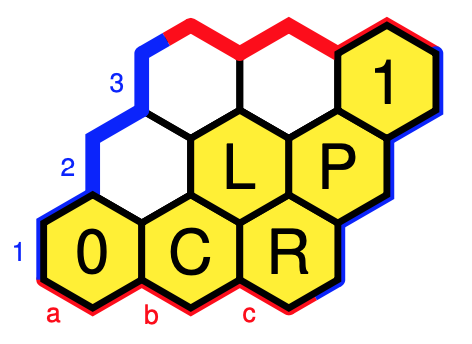In this case, Grothendieck topologies coincide with Lawvere-Tierney topologies, which come from closure operators $j~:~\Omega \rightarrow \Omega$ which are order-increasing, idempotent, and compatible with the $T$-action and with the $\wedge$, that is,

• if $A \leq B$, then $j(A) \leq j(B)$
• $j(j(A)) = j(A)$
• $j(A).t=j(A.t)$
• $j(A \wedge B) = j(A) \wedge j(B)$

Colouring all cells with the same $j$-value alike, and remaining cells $A$ with $j(A)=A$ coloured yellow, we have six such closure operations $j$, that is, Grothendieck topologies.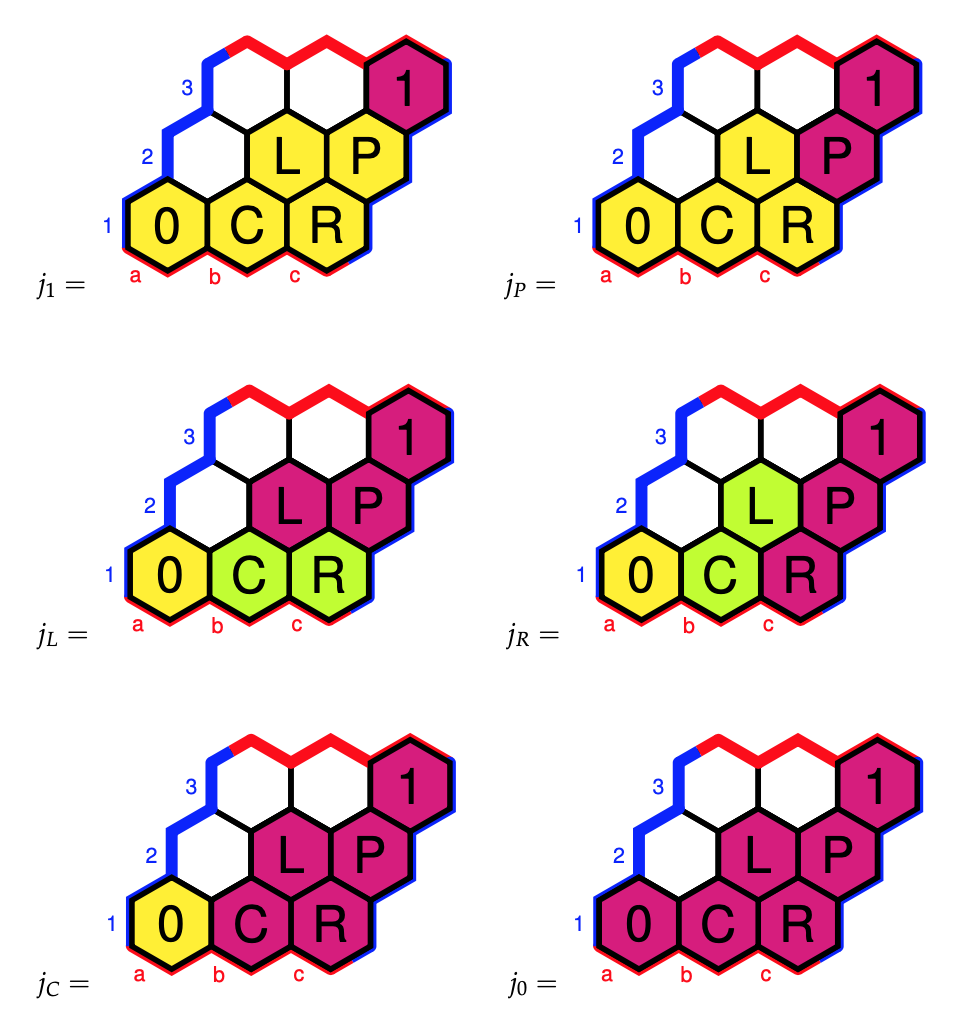The triadic monoid $T$ acts via affine transformations on the set of pitch classes $\mathbb{Z}_{12}$ and we’ve defined it such that it preserves the notes $\{ 0,4,7 \}$ of the major $(0,4,7)$-chord, that is, $\{ 0,4,7 \}$ is a subobject of $\mathbb{Z}_{12}$ in the topos of $T$-sets.

The point of the subobject classifier $\Omega$ is that morphisms to it classify subobjects, so there must be a $T$-equivariant map $\chi$ making the diagram commute (vertical arrows are the natural inclusions)
$\xymatrix{\{ 0,4,7 \} \ar[r] \ar[d] & 1 \ar[d] \\ \mathbb{Z}_{12} \ar[r]^{\chi} & \Omega}$

What does the morphism $\chi$ do on the other pitch classes? Well, it send an element $k \in \mathbb{Z}_{12} = \{ 1,2,\dots,12=0 \}$ to

• $1$ iff $k \in \{ 0,4,7 \}$
• $P$ iff $a(k)$ and $b(k)$ are in $\{ 0,4,7 \}$
• $L$ iff $a(k) \in \{ 0,4,7 \}$ but $b(k)$ is not
• $R$ iff $b(k) \in \{ 0,4,7 \}$ but $a(k)$ is not
• $C$ iff neither $a(k)$ nor $b(k)$ is in $\{ 0,4,7 \}$

Remember that $a$ and $b$ are the transformations (images of $(1,2,\dots,12)$)
 a:=Transformation([10,1,4,7,10,1,4,7,10,1,4,7]);; b:=Transformation([12,8,4,12,8,4,12,8,4,12,8,4]);; 
so we see that

• $0,1,4$ are mapped to $1$
• $3$ is mapped to $P$
• $8,11$ are mapped to $L$
• $1,6,9,10$ are mapped to $R$
• $2,5$ are mapped to $C$

Finally, we can compute the sheafification of the sub-presheaf $\{ 0,4,7 \}$ of $\mathbb{Z}$ with respect to a Grothendieck topology $j$: it consists of the set of those $k \in \mathbb{Z}_{12}$ such that $j(\chi(k)) = 1$.

The musically interesting Grothendieck topologies are $j_P, j_L$ and $j_R$ with corresponding sheaves:

• For $j_P$ we get the sheaf $\{ 0,3,4,7 \}$ which Mamuth-ers call a Major-Minor Mixture as these are the notes of both the major and minor $0$-triads
• For $j_L$ we get $\{ 0,3,4,7,8,11 \}$ which is an example of an Hexatonic scale (six notes), here they are the notes of the major and minor $0,~4$ and $8$-triads
• For $j_R$ we get $\{ 0,1,3,4,6,7,9,10 \}$ which is an example of an Octatonic scale (eight notes), here they are the notes of the major and minor $0,~3,~6$ and $9$-triads

We could have played the same game starting with the three notes of any other major triad.

Those in the know will have noticed that so far I’ve avoided another incarnation of the dihedral $D_{12}$ group in music, namely the $PLR$-group, which explains the notation for the elements of the subobject classifier $\Omega$, but this post is already way too long.

(to be continued…)

A couple of days ago, Peter Rowlett posted on The Aperiodical: Introducing hexboard – a LaTeX package for drawing games of Hex.

Hex is a strategic game with two players (Red and Blue) taking turns placing a stone of their color onto any empty space. A player wins when they successfully connect their sides together through a chain of adjacent stones.

Here’s a short game on a $5 \times 5$ board (normal play uses $11\times 11$ boards), won by Blue, drawn with the LaTeX-package hexboard.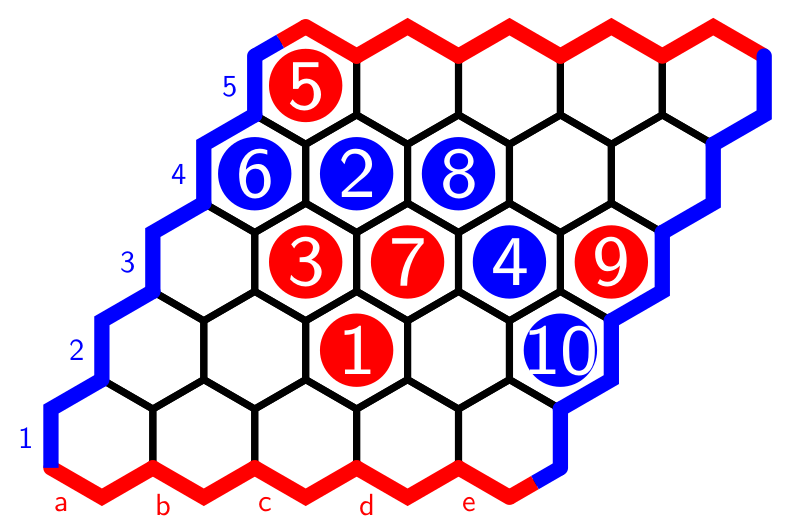As much as I like mathematical games, I want to use the versability of the hexboard-package for something entirely different: drawing finite Heyting algebras in which it is easy to visualise the logical operations.

Every full hexboard is a poset with minimal cell $0$ and maximal cell $1$ if cell-values increase if we move horizontally to the right or diagonally to the upper-right. With respect to this order, $p \vee q$ is the smallest cell bigger than both $p$ and $q$, and $p \wedge q$ is the largest cell smaller than $p$ and $q$.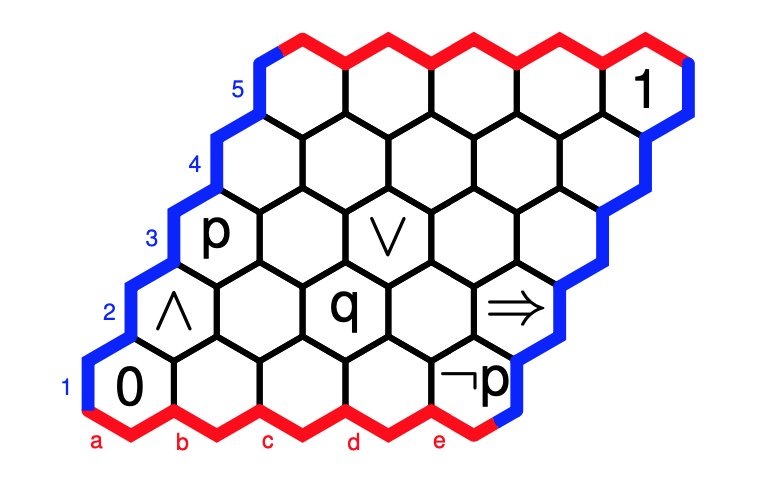The implication $p \Rightarrow q$ is the largest cell $r$ such that $r \wedge p \leq q$, and the negation $\neg p$ stands for $p \Rightarrow 0$. With these operations, the full hexboard becomes a Heyting algebra.

Now the fun part. Every filled area of the hexboard, bordered above and below by a string of strictly increasing cells from $0$ to $1$ is also a Heyting algebra, with the induced ordering, and with the logical operations defined similarly.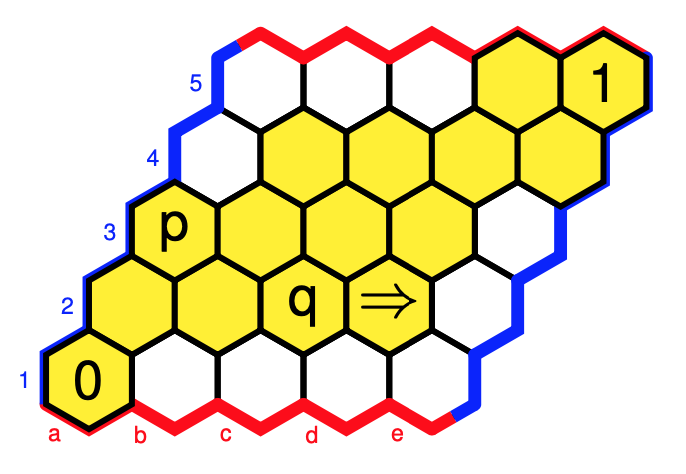Note that this mustn’t be a sub-Heyting algebra as the operations may differ. Here, we have a different value for $p \Rightarrow q$, and $\neg p$ is now $0$.

If you’re in for an innocent “Where is Wally?”-type puzzle: $W = (\neg \neg p \Rightarrow p)$.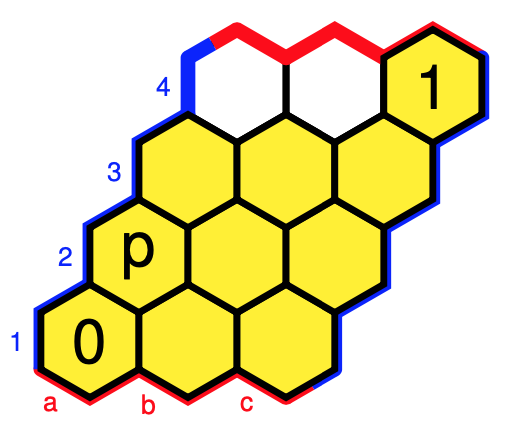Click on the image to get the solution.

The downsets in these posets can be viewed as the open sets of a finite topology, so these Heyting algebra structures come from the subobject classifier of a topos.

There are more interesting toposes with subobject classifier determined by such hex-Heyting algebras.

For example, the Topos of Triads of Thomas Noll in music theory has as its subobject classifier the hex-Heyting algebra (with cell-values as in the paper):Note to self: why not write a couple of posts on this topos?

Another example: the category of all directed graphs is the presheaf topos of the two object category ($V$ for vertices, and $E$ for edges) with (apart from the identities) just two morphisms $s,t : V \rightarrow E$ (for start- and end-vertex of a directed edge).

The subobject classifier $\Omega$ of this topos is determined by the two Heyting algebras $\Omega(E)$ and $\Omega(V)$ below.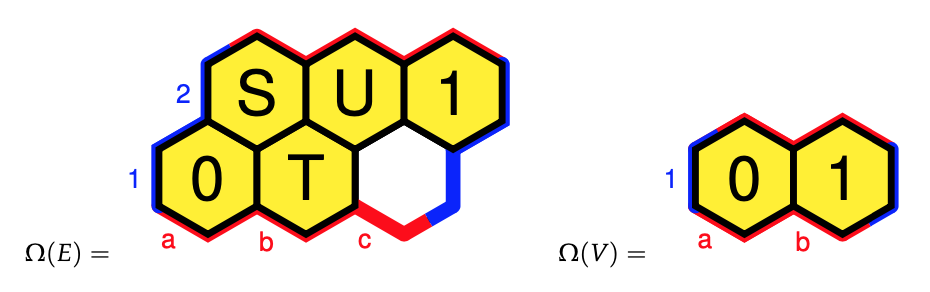These ‘hex-Heyting algebras’ are exactly what Eduardo Ochs calls ‘planar Heyting algebras’.

Eduardo has a very informative research page, containing slides and handouts of talks in which he tries to explain topos theory to “children” (using these planar Heyting algebras) including:

Perhaps now is a good time to revive my old sga4hipsters-project.

Raymond Smullyan‘s logic puzzles frequently involve Knights (who always tell the truth) and Knaves (who always lie).

In his book Logical Labyrinths (really a first course in propositional logic) he introduced islands where the lying or truth-telling habits can vary from day to day—that is, an inhabitant might lie on some days and tell the truth on other days, but on any given day, he or she lies the entire day or tells the truth the entire day.

An island is said to be Boolean if is satisfies the following conditions:

• $\mathbf{N}$ : For any inhabitant $A$ there is an inhabitant who tells the truth on all and only those days on which $A$ lies.
• $\mathbf{M}$ : For any inhabitants $A$ and $B$ there is an inhabitant $C$ who tells the truth on all and only those days on which $A$ and $B$ both tell the truth.
• $\mathbf{J}$ : For any inhabitants $A$ and $B$ there is an inhabitant $C$ who tells the truth on all and only those days on which either $A$ tells the truth or $B$ tells the truth (or both). (In other words, $C$ lies on those and only those days on which $A$ and $B$ both lie.)

On any given day there are only Knights and Knaves on the island, but these two populations may vary from one day to the other. The subsets (of all days) for which there is an inhabitant who is a Knight then and a Knave on all other days form a Boolean algebra with operations $\wedge = \cap$ ($\mathbf{M}$eet), $\vee= \cup$ ($\mathbf{J}$oin) and $\neg=$ set-complement ($\mathbf{N}$egation).

Here’s a nice puzzle from Smullyan’s book:

Solomon’s Island also turned out to be quite interesting. When Craig arrived on it, he had the following conversation with the resident sociologist:

Craig : Is this island a Boolean island?
Sociologist : No.
Craig : Can you tell me something about the lying and truth-telling habits of the residents here?
Sociologist : For any inhabitants $A$ and $B$, there is an inhabitant $C$ who tells the truth on all and only those days on which either $A$ lies or $B$ lies (or both).

Show that the sociologist didn’t go native, and that his research is lousy.
(My wording, not Smullyan’s)

Smullyan’s version: This interview puzzled inspector Craig; he felt that something was wrong. After a while he realized for sure that something was wrong, the sociologist was either lying or mistaken!

Extending Smullyan’s idea, we can say that an island is Heyting if, in addition to $\mathbf{M}$ and $\mathbf{J}$ is satisfies the following rules

• $\mathbf{T}$ : at least one inhabitant tells the truth on all days.
• $\mathbf{F}$ : at least one inhabitant lies on all days.
• $\mathbf{I}$ : For any inhabitants $A$ and $B$ there is an inhabitant $C$ sharing Knight-days with $A$ only when $B$ tells the truth, and there are no inhabitants doing this while telling the truth on more days than $C$.

Let’s give an example of an Heyting island which is not Boolean.

On Three-island there are only three kinds of people: Knights, Knaves and Alternates, who can neither lie nor tell the truth two days in a row. All Alternates tell the truth on the same days.

Here’s a riddle:

You meet John, who is a Knight, James, an Alternate, and William, a Knave. You don’t know who is who. You can only ask one question containing at most four words, giving you a Yes or No answer, to just one of the three. The answer must tell you whether that person is James or not.

You may like to watch Smullyan on the Carson show for a hint.

Or, you might just watch it reminiscing long forgotten times, when talkshow-hosts still listened to their guests, and could think for themselves…

In this neverending pandemic there’s a shortage of stories putting a lasting smile on my face. Here’s one.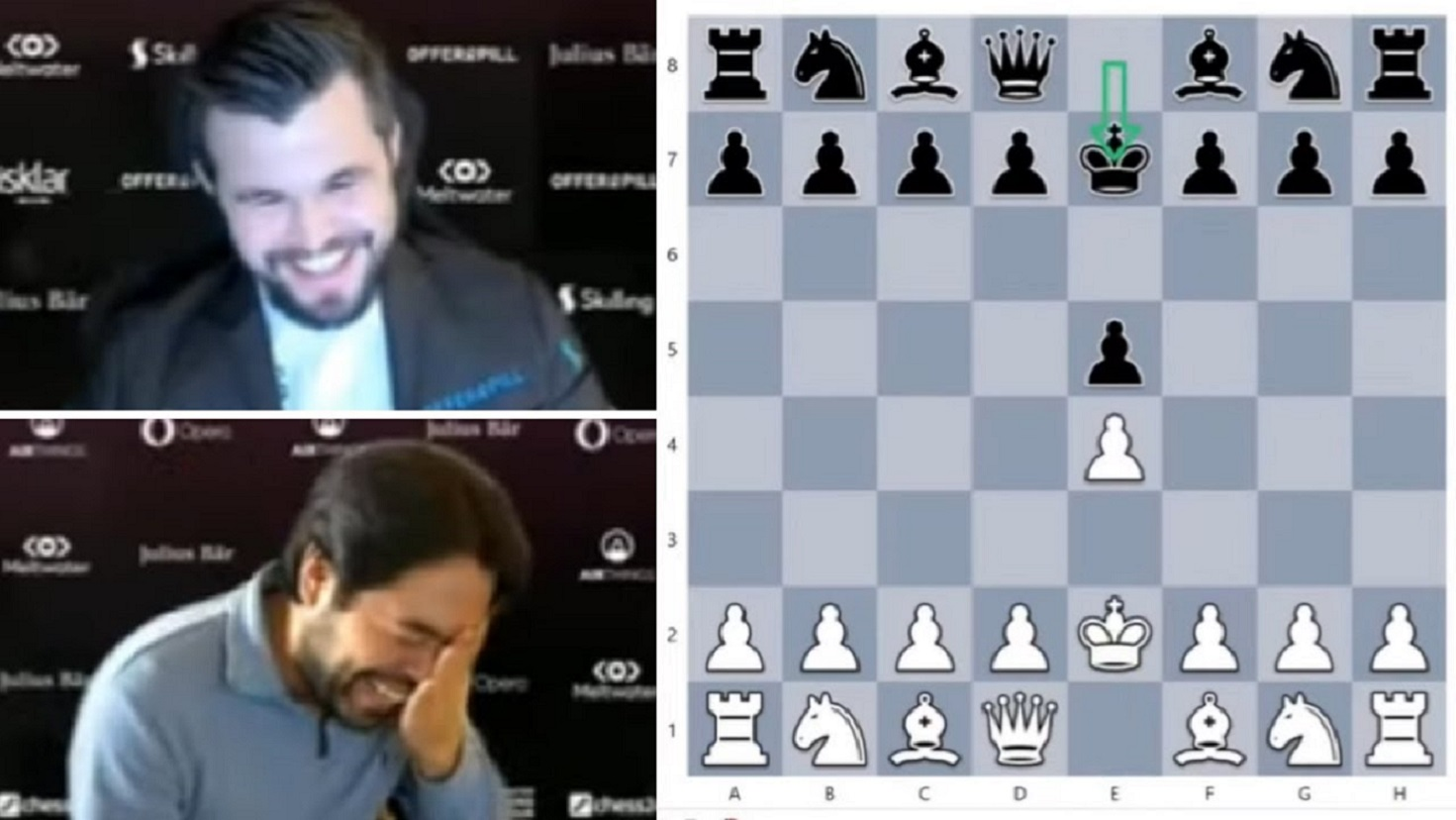If you are at all interested in chess, you’ll know by now that some days ago IGMs (that is, international grandmasters for the rest of you) Magnus Carlsen and Hikaru Nakamura opened an official game with a double bongcloud, and couldn’t stop laughing.

The bongcloud attack is the chess opening in which white continues after

1. e2-e4, e7-e5

with

2. Ke1-e2 !

thereby blocking the diagonals for the bishop and queen, losing the ability to castle, and putting its king in danger.

If you are left clueless, you should download the free e-book Winning with the bongcloud immediately.

If you are (or were) a chess player, it is the perfect parody to all those books you had to suffer through in order to build up an ‘opening repertoire’.

If you are new to chess (perhaps after watching The queen’s gambit), it gives you a nice selection of easy mate-in-one problems.

Every possible defence against the bongcloud is illustrated with a ‘game’ illustrating the massive advantage the attack gives, ending with a situation in which … black(!) has a one-move mate.

One example:

In the two knight Copacabana tango defense against the bongcloud, that is the position after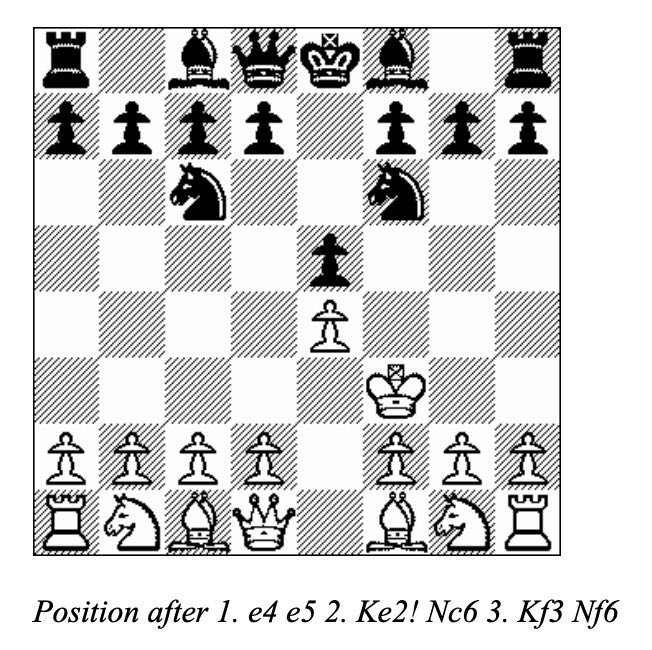the Haight-Asbury (yeah, well) game (Linares, 1987) continued with:

4. Kg3, Nxe4?
5. Kh3, d6+
6. Qg4!, Bxg4
7. Kxg4, Qf6??
8. Ne2, h5+??
9. Kh3!, Nxf2
10. Kg3!

giving this position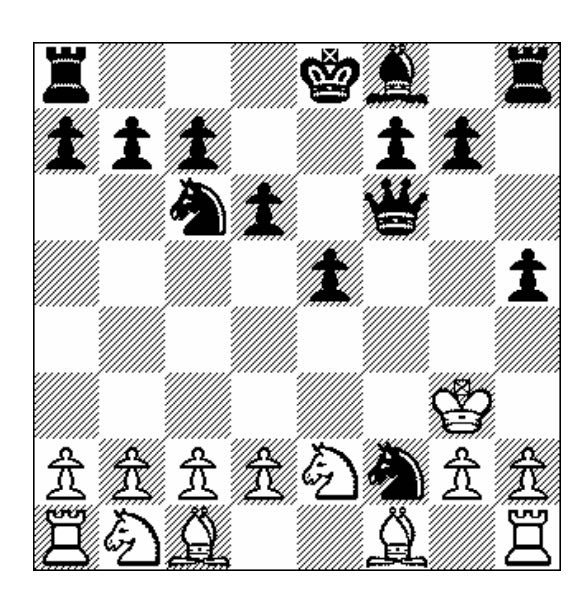which ‘Winning with the bongcloud’ evaluates as:

White continues his textbook execution of a “pendulum,” swinging back and forth between g3 and h3 to counter every Black threat. With the dynamically placed King, Black’s attack teeters on the edge of petering out. Nxh1 will trap the Black Knight and extinguish the threat.

In the actual game, play took a different turn as Black continued his h-file pressure. Nonetheless, this game is an excellent example of how 2. …Nc6 is often a wasted tempo in the Bongcloud.

Here’s Nakamura philosophising over the game and the bongcloud. Try to watch at least the first 30 seconds or so to see the commentators reaction to the actual Carlsen-Nakamura game.

Now, that put a smile on your face, didn’t it?

Around 1975 Sir Roger Penrose discovered his aperiodic P2 tilings of the plane, using only two puzzle pieces: Kites (K) and Darts (D)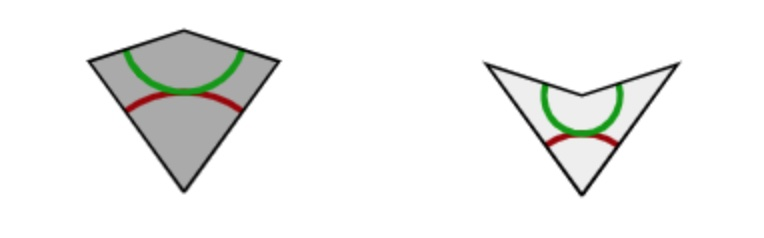The inner angles of these pieces are all multiples of $36^o = \tfrac{180^o}{5}$, the short edges have length $1$, and the long edges have length $\tau = \tfrac{1+\sqrt{5}}{2}$, the golden ratio. These pieces must be joined together so that the colours match up (if we do not use this rule it is easy to get periodic tilings with these two pieces).

There is plenty of excellent online material available:

• The two original Martin Gardner Scientific American articles on Penrose tiles have been made available by the MAA, and were reprinted in “Penrose Tiles to Trapdoor Ciphers”. They contain most of Conway’s early discoveries about these tilings, but without proofs.
• A JavaScript application by Kevin Bertman to play around with these tilings. You can deflate and inflate tilings, find forced tiles and much more. Beneath the app-window there’s a detailed explanation of all the basics, including inflation and deflation of the P2-tiles, the seven types of local vertex configurations (naming by Conway, of course),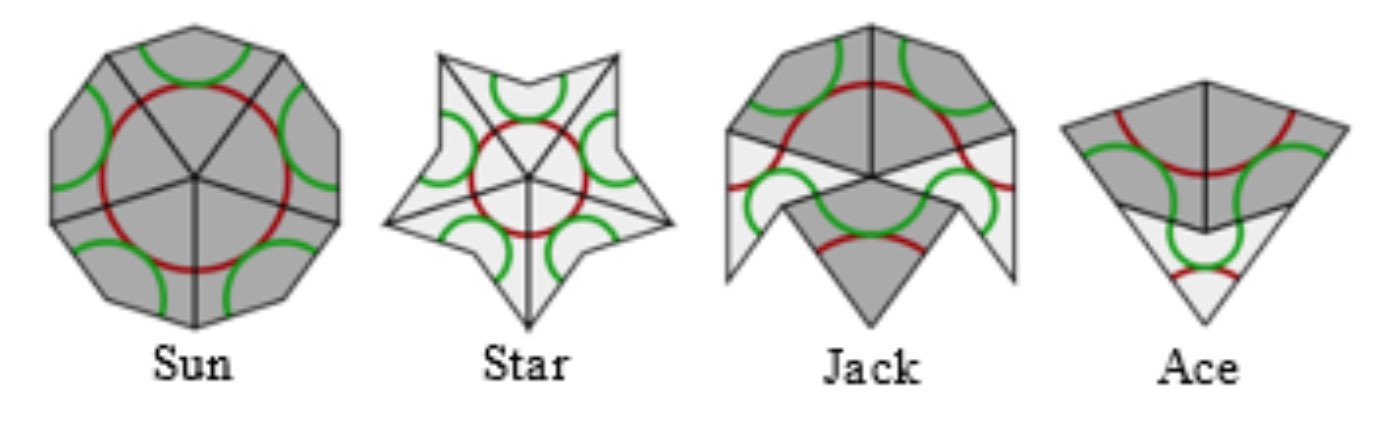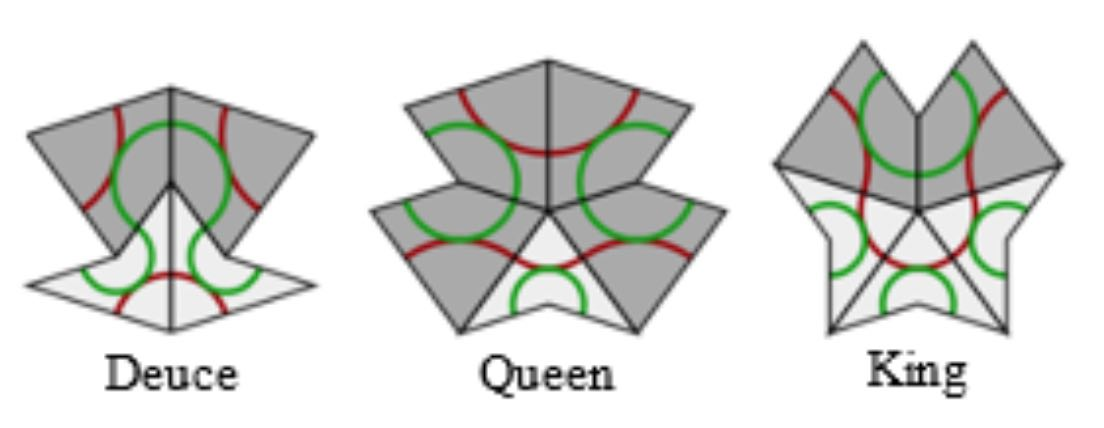proofs of aperiodicity (similar to the one for Conway’s musical sequences), that every tile lies within an ace (similar to the LSL-subword in musical sequences) with application to local isomorphism (again similar to the $1$-dimensional case).
• Course notes of an Oxford masterclass in geometry Lectures on Penrose tilings by Alexander Ritter, again with proofs of all of the above and a discussion about the Cartwheel tilings (similar to that in the post on musical sequences), giving an algorithm to decide whether or not a partial tiling can be extended to the entire plane, or not.

There’s no point copying this material here. Rather, I’d like to use some time in this GoV series of posts to talk about de Bruijn’s pentagrid results. For this reason, I now need to make the connection with Penrose’s ‘other’ tilings, the P3 tiles of ‘thin’ and ‘thick’ rhombi (sometimes called ‘skinny’ and ‘fat’ rhombi).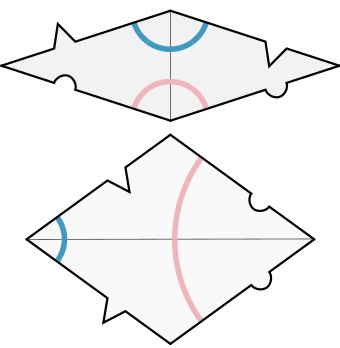Every Penrose P2-tiling can be turned into a P3-rhombic tiling, and conversely.

From kites and darts to rhombi: divide every kite in two halves along its line of reflection. Then combine darts and half-kites into rhombi where a fat rhombus consists of a dart and two half-kites, joined at a long edge, and a skinny rhombus is made of two half-kites, joined at a short edge. This can always be done preserving the gluing conditions. It suffices to verify this for the deflated kite and dart (on the left below) and we see that the matching colour-conditions are those of the rhombi.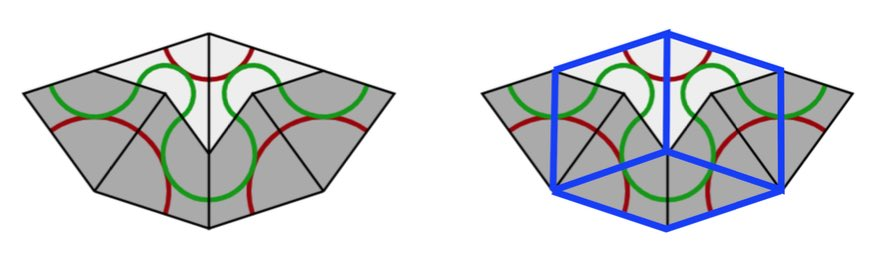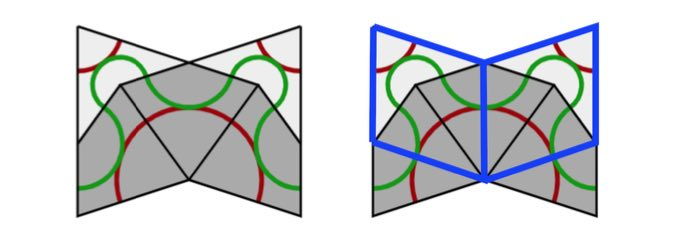From rhombi to kites and darts: divide every fat rhombus into a dart (placed at the red acute angle) and two half-kites, joined at a long edge, and divide every skinny rhombus into two half-kites along its short diagonal.

All results holding for Kites and Darts tilings have therefore their versions for Rhombic tilings. For example, we have rhombic deflation and inflation rules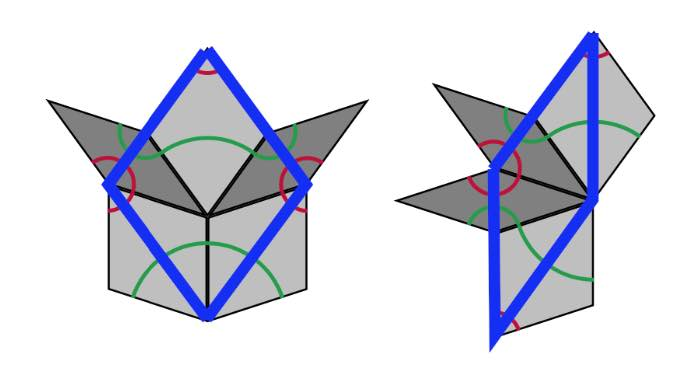A Rhombic tiling can be seen as an intermediate step in the inflation process of a Penrose tiling $P$. Start by dividing all kites in two halves along their long diagonal and all darts in two halves along their short diagonal (the purple lines below).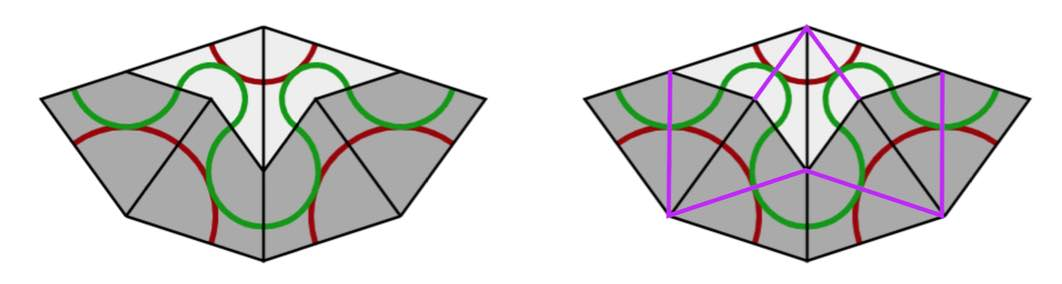If we consider all original black lines together with the new purple ones, we get a tiling of the plane by triangles and we call this the $A$-tiling. There are two triangles, s small triangle $S_A$ (the whiter ones, with two small edges ofd length $1$ and one edge of length $\tau$) and a large triangle $L_A$ (the greyer ones, with two edges of length $\tau$ and one edge of length $1$).

Next, we remove the black lines joining an $S_A$-triangle with an $L_A$-triangle, and get another tiling with triangles, the $B$-tiling, with two pieces, a large triangle $L_B$ with two sides of length $\tau$ and one side of length $1+\tau$ (the white ones) (the union of an $L_A$ and a $S_A$), and a small triangle $S_B$ which has the same form as $L_A$ (the greyer ones). If we now join two white triangles $L_B$ along their common longer edge, and two greyer triangles $S_B$ along their common smaller edge, we obtain the Rhombic tiling $R$ corresponding to $P$.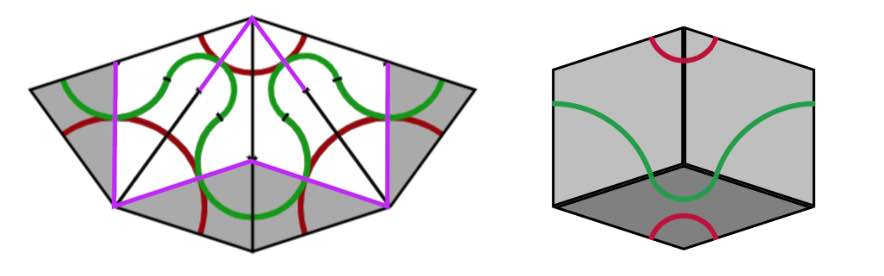We can repeat this process starting with the Rhombic tiling $R$. We divide all fat rhombi in two along their long diagonals, and the skinny rhombi in two along their short diagonals (the purple lines). We obtain a tiling of the plane by triangles, which is of course just the $B$-tiling above.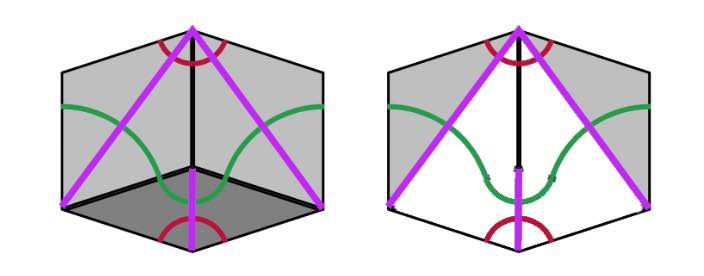Remove the edge joining a small $S_B$ with a large $L_B$ triangle, then we get a new tiling by triangles, the $\tau A$-tiling consisting of large triangles $L_{\tau A}$ (the white ones) with two long edges of length $1+\tau$ and one short edge of length $\tau$ (note that $L_{\tau A}$ is $\tau$ times the triangle $L_A$), and a smaller one $S_{\tau A}$ (the grey ones) having two short edges of length $\tau$ and one long edge of length $1+\tau$ (again $S_{\tau A}$ is $\tau$ times the triangle $S_A$). If we join two $L_{\tau A}$-triangles sharing a common long edge we obtain a Kite ($\tau$-times larger than the original Kite) and if we joint two $S_{\tau A}$-triangles along their common small edge we get a Dart ($\tau$ times larger than the original Dart). The Penrose tiling we obtain is the inflation $inf(P)$ of the original $P$.

If we repeat the whole procedure starting from $inf(P)$ instead of $P$ we get, in turn, triangle tilings of the plane, subsequently the $\tau B$-tiling, the $\tau^2 A$-tiling, the $\tau^2 B$-tiling and so on, the triangles in a $\tau^n A$-tiling being of size $\tau^2$-times those of the $A$-tiling, and those in the $\tau^n B$-tiling $\tau^n$-times as large as those of the $B$-tiling.

The upshot of this is that we can associate to a Penrose tiling $P$ of the plane a sequence of $0$’s and $1$’s. Starting from $P$ we have an infinite sequence of associated triangle tilings
$A,~B,~\tau A,~\tau B,~\tau^2 A, \dots,~\tau^n A,~\tau^n B,~\tau^{n+1} A, \dots$
with larger and larger triangle tiles. Let $p$ be a point of the plane lying in the interior of an $A$-tile, then we define its index sequence
$i(P,p) = (x_0,x_1,x_2,\dots ) \quad x_{2n} = \begin{cases} 0~\text{if p \in L_{\tau^n A}} \\ 1~\text{if p \in S_{\tau^n A}} \end{cases}~ x_{2n+1} = \begin{cases} 0~\text{if p \in L_{\tau^n B}} \\ 1~\text{if p \in S_{\tau^n B}} \end{cases}$
That is, $x_0=1$ if $p$ lies in a small triangle of the $A$-tiling and $x_0=1$ if it lies in a large triangle, $x_1=1$ if $p$ lies in a small triangle of the $B$-tiling and is $0$ if it lies in a large $B$-triangle, and so on.

The beauty of this is that every infinite sequence $(x_0,x_1,x_2,\dots )$ whose terms are $0$ or $1$, and in which no two consecutive terms are equal to $1$, is the index sequence $i(P,p)$ of some Penrose tiling $P$ in some point $p$ of the plane.

From the construction of the sequence of triangle-tilings it follows that a small triangle is part of a large triangle in the next tiling. For this reason an index sequence can never have two consecutive ones. An index sequence gives explicit instructions as to how the Penrose tiling is constructed. In each step add a large or small triangle as to fit the sequence, together with the matching triangle (the other half of a Penrose or Rhombic tile). Next, look at the patches $P_i$ of Kites and Darts (in the $\tau^i A$ tiling), for $i=0,1,\dots$, then $P_{i-k}$ is contained in the $k$-times inflated $P_i$, $i^k(P_i)$ (without rescaling), for each $i$ and all $1 \leq k \leq i$. But then, we have a concentric series of patches
$P_0 \subset i(P_1) \subset i^2(P_2) \subset \dots$
filling the entire plane.The index sequence depends on the choice of point $p$ in the plane. If $p’$ is another point, then as the triangle tiles increase is size at each step, $p$ and $p’$ will lie in the same triangle-tile at some stage, and after that moment their index sequences will be the same.

As a result, there exists an uncountable infinity of distinct Penrose tilings of the plane.

From the discussions we see that a Penrose tiling is determined by the index-sequence in any point in the plane $(x_0,x_1,\dots )$ consisting of $0$’s and $1$’s having no two consecutive $1$’s, and that another such sequence $(x’_0,x’_1,\dots )$ determines the same Penrose tilings if $x_n=x’_n$ for all $n \geq N$ for some number $N$. That is, the Penrose tiling is determined by the the equivalence class of such sequences, and it is easy to see that there are uncountably many such equivalence classes.

If you want to play a bit with Penrose tiles, you can order the P2 tiles or the P3 tiles from
Cherry Arbor Design.

“A subway is just a hole in the ground, and that hole is a maze.”

“The map is the last vestige of the old system. If you can’t read the map, you can’t use the subway.”

Eddie Jabbour in Can he get there from here? (NYT)

Sometimes, lines between adjacent stations can be uni-directional (as in the Paris Metro map below in the right upper corner, 7bis). So, it is best to view a subway map as a directed graph, with vertices the different stations, and directed arrows when there’s a service connecting two adjacent stations.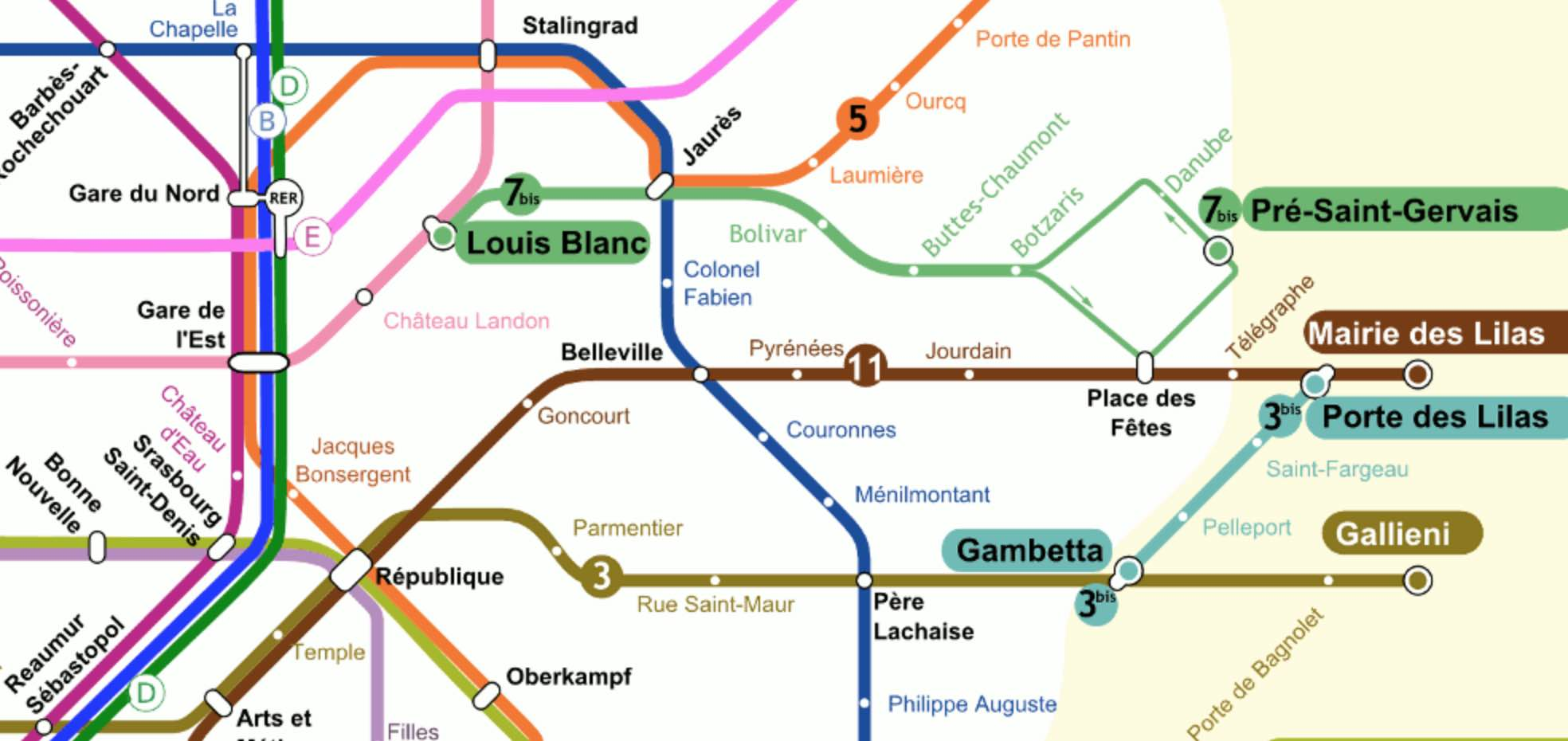Aha! But, directed graphs form a presheaf topos. So, each and every every subway in the world comes with its own logic, its own bi-Heyting algebra!

Come again…?

Let’s say Wally (or Waldo, or Charlie) is somewhere in the Paris metro, and we want to find him. One can make statements like:

$P$ = “Wally is on line 3bis from Gambetta to Porte des Lilas.”, or

$Q$ = “Wally is traveling along line 11.”

Each sentence pinpoints Wally’s location to some directed subgraph of the full Paris metro digraph, let’s call this subgraph the ‘scope’ of the sentence.

We can connect such sentences with logical connectives $\vee$ or $\wedge$ and the scope will then be the union or intersection of the respective scopes.

The scope of $P \vee Q$ is the directed subgraph of line 11 (in both directions) together with the directed subgraph of line 3bis from Gambetta to Porte des Lilas.

The scope of $P \wedge Q$ is just the vertex corresponding to Porte des Lilas.

The scope of the negation $\neg R$ of a sentence $R$ is the subgraph complement of the scope of $R$, so it is the full metro graph minus all vertices and directed edges in $R$-scope, together with all directed edges starting or ending in one of the deleted vertices.

For example, the scope of $\neg P$ does not contain directed edges along 3bis in the reverse direction, nor the edges connecting Gambetta to Pere Lachaise, and so on.

In the Paris metro logic the law of double negation does not hold.$\neg \neg P \not= P$ as both statements have different scopes. For example, the reverse direction of line 3bis is part of the scope of $\neg \neg P$, but not of $P$.

So, although the scope of $P \wedge \neg P$ is empty, that of $P \vee \neg P$ is not the full digraph.

The logical operations $\vee$, $\wedge$ and $\neg$ do not turn the partially ordered set of all directed subgraphs of the Paris metro into a Boolean algebra structure, but rather a Heyting algebra.

Perhaps we were too drastic in removing all “problematic edges” from the scope of $\neg R$ (those with a source or target station belonging to the scope of $R$)?

We might have kept all problematic edges, and added the missing source and/or target stations to get the scope of another negation of $R$: $\sim R$.

Whereas the scope of $\neg \neg R$ always contains that of $R$, the scope of $\sim \sim R$ is contained in $R$’s scope.

The scope of $R \vee \sim R$ will indeed be the whole graph, but now $R \wedge \sim R$ does no longer have to be empty. For example, $P \wedge \sim P$ has as its scope all stations on line 3bis.

In general $R \wedge \sim R$ will be called the ‘boundary’ $\partial(R)$ of $R$. It consists of all stations within $R$’s scope that are connected to the outside of $R$’s scope.

The logical operations $\vee$, $\wedge$, $\neg$ and $\sim$ make the partially ordered set of all directed subgraphs of the Paris metro into a bi-Heyting algebra.

There’s plenty more to say about all of this (and I may come back to it later). For the impatient, there’s the paper by Reyes and Zolfaghari: Bi-Heyting Algebras, Toposes and Modalities.

Right now, I’m more into exploring whether this setting can be used to revive an old project of mine: Heyting Smullyanesque problems (btw. the algebra in that post is not Heyting, oops!).

Raymond Smullyan brought Knights and Knaves puzzles to a high art in his books. Here’s the setting:

On Smullyan’s islands there are Knights, who always tell true statements, Knaves, who always lie, and sometimes also Normals, who sometimes tell the truth and sometimes lie.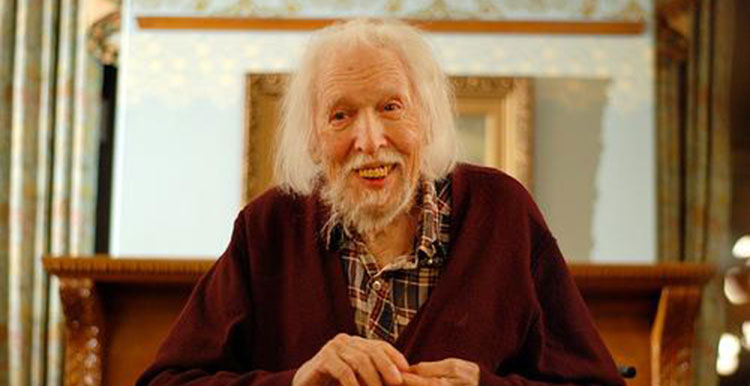(image credit MikeKlein)

Problems of this sort can be solved by classical propositional logic, replacing every sentence $P$ told by inhabitant $A$ by the formula $k_A \leftrightarrow P$ where $k_A$ is the sentence “A is a Knight”.

Some time ago I asked for Smullyanesque problems in a non-classical logic, where we replace the usual truth-values $T$ (true) and $F$ (false) of propositional logic by elements of an Heyting algebra.

Jason Rosenhouse wrote a paper Knights, Knaves, Normals, and Neutrals for The College Mathematics Journal, containing more information than in his blog post, as well as some nice challenging puzzles. Here’s Rosenhouse’s setting:

Apart from Knights, Knaves and Normals there’s also the tribe of Neutrals (which he describes as a sort of trans-Knights or trans-Knaves) who can only tell sentences with truth value $N$ in the Heyting algebra $\{ T,N,F \}$ with logical connectives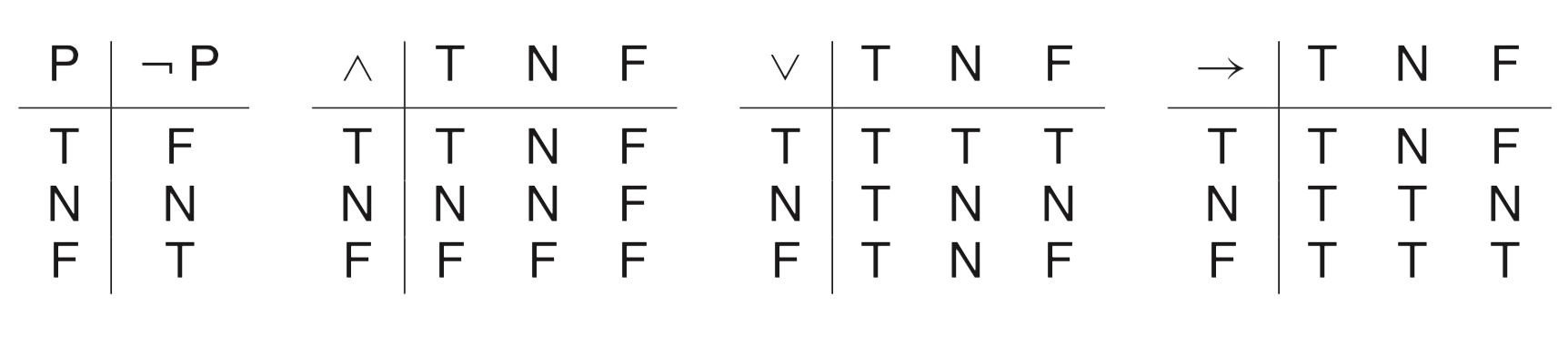A typical sentence of truth value $N$ is “A is a Knight” or “A is a Knave” whereas, in reality, $A$ is Neutral. Here are the truth values of sentences about a person (the column headings) with respect to the actual situation (the row headings)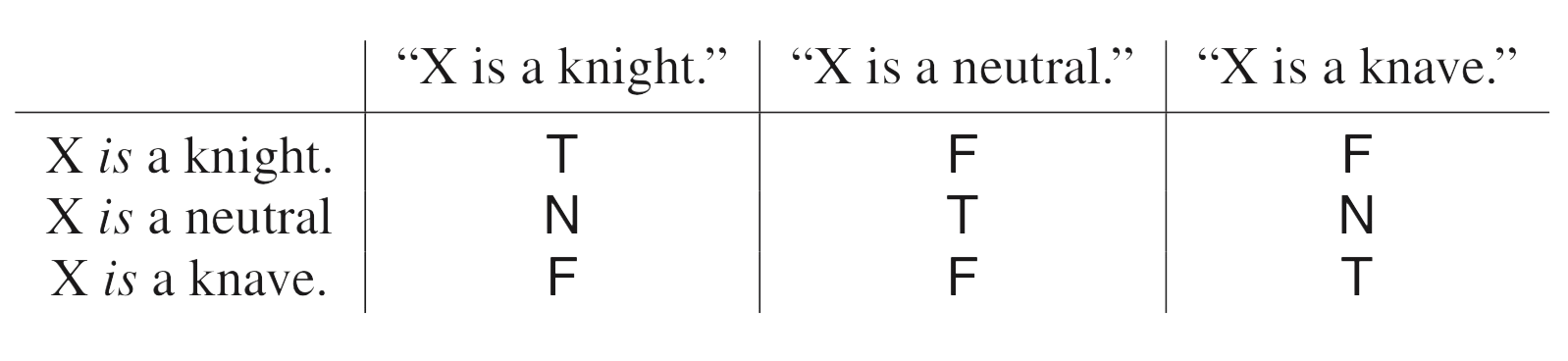A good way into such problems is to focus on sentence like “A is a Neutral” as they have only classical truth values $T$ or $F$ and to remember that Neutrals can never say a sentence with classical truth value. Here’s an example (only Knights,Knaves or Neutrals present):

Problem 1: Suppose you meet three people, named Dave, Evan and Ford. They make the following statements:

Dave: Evan is a knight.
Evan: Ford is a knave.
Ford: Dave is a neutral.

Can you determine the types of all three people?

Solution: Ford’s sentence has value $T$ or $F$, so Ford cannot be Neutral so must be a Knight or Knave. But then Evan’s sentence also has classical truth value, so he can’t be Neutral either, and by the same reasoning also Dave cannot be neutral. So, we know that all three people must be either Knights or Knaves and then it follows at once that Ford is a Knave (because he lied) and that Evan and Dave must be knights.

These puzzles become more interesting once we use logical connectives.

Problem 2: What can you conclude from this dialog?

Mimi: Olaf is a Knight and Olaf is not a Knight.
Nate: Olaf is a Knave or Olaf is not a Knave.
Olaf: Mimi is a Knight or Nate is a Knave or I am Neutral.

Solution: Mimi’s sentence can only have truth value $F$ or $N$ so she can’t be a Knight. Nate’s sentence has value $T$ or $N$ so he cannot be a Knave.
The two first parts of Olaf’s line cannot be $T$ so must be either $N$ or $F$. So, Olav’s line has total value $T$ only if the last part ‘I am Neutral’ is $T$. So, Olaf can neither be a Knight (because then the last part is $F$) nor Neutral (because then the line would have value $T$ and Neutrals can only speak $N$-sentences). So, Olaf must be a knave. Nate must then be a Knight and Mimi a Knave (because the first part of her line is $F$ and so must be the whole sentence).

The situation becomes even more complicated when we allow Normals (who sometimes lie and sometimes tell the truth but never say sentences with value $N$) to be present. Here’s Rosenhouse’s ‘Grand Finale’:

Problem 3: One day a visitor encountered eight people, among them exactly two Knights, two Knaves, two Normals and two Neutrals. What can you conclude from this dialog:

Sara: Walt is a Neutral or Vera is a Neutral.
Todd: Xara is a Knave and Yoav is a Knave.
Ursa: If Sara is a Knight, then Todd and Yoav are Normals.
Vera: I am not a Neutral.
Walt: If Vera is not a Neutral, then neither is Todd.
Xara: Todd is a Knight if and only if Zack is a Neutral.
Zack: Xara is a Neutral if and only if Walt is not a Normal.

You will solve this problem much quicker than to read through the long explanation in Rosenhouse’s paper.

These puzzles beg to be generalised to more complicated Heyting algebras.

What about a book on “Knights, Knaves and Knols”? A Knol (or $X$-Knol) has knowledge limited to sentences with truth value $X$, an element in the Heyting algebra.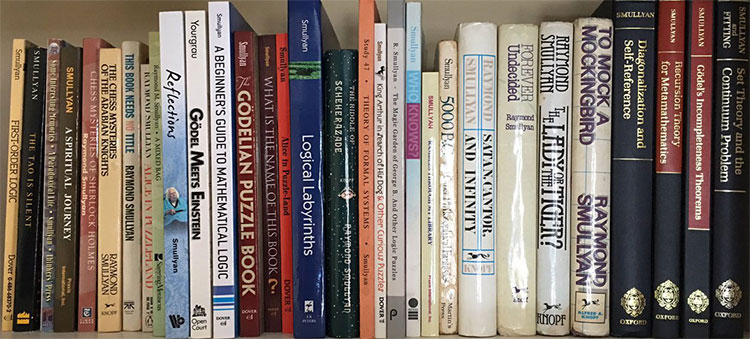(image credit: Joe Blitzstein via Twitter)

Smullyan’s Knights and Knaves problems are classics. On an island all inhabitants are either Knights (who only tell true things) and Knaves (who always lie). You have to determine their nature from a few statements. Here’s a very simple problem:

“Abercrombie met just two inhabitants, A and B. A made the following statement: “Both of us are Knaves.” What is A and what is B?”

Now, this one is simple enough to solve, but for more complicated problems a generic way to solve the puzzles is to use propositional calculas, as explained in Smullyan’s Logical Labyrinths”, chapter 8 “Liars, truth-tellers and propositional logic’.

If an inhabitants $A$ asserts a proposition $P$, and if $k_A$ is the assertion ‘$A$ is a Knight’, then the statement can be rephrased as

$k_A \Leftrightarrow P$

for if $A$ is a Knight, $P$ must be true and if $A$ is a Knave $P$ must be false.

Usually, one can express $P$ as a propositional statement involving $k_A,k_B,k_C,\dots$.
The example above can be rephrased as

$k_A \Leftrightarrow (\neg k_A \wedge \neg k_B)$

Assigning truth values to $k_A$ and $k_B$ and setting up the truth-table for this sentence, one sees that the only possibility for this to be true is that $k_A$ equals $0$ and $k_B$ equals $1$. So, $A$ is a Knave and $B$ is a Knight.

Clearly, one only requires this approach for far more difficult problems.

In almost all Smullyan puzzles, the only truth values are $0$ and $1$. There’s a short excursion to Boolean algebras (sorry, Boolean islands) in chapter 9 ‘Variable Liars’ in Logical Labyrinths. But then, the type of problems are about finding equivalent notions of Boolean algebras, rather that generalised Knights&Knaves puzzles.

Did anyone pursue the idea of Smullyanesque puzzles with truth values in a proper Heyting algebra?

I only found one blog-post on this: Non-Classical Knights and Knaves by Jason Rosenhouse.

He considers three valued logic (the Heyting algebra corresponding to the poset 0-N-1, and logical connectives as in the example on the Wiki-page on Heyting algebras.

On his island the natives cycle, repeatedly and unpredictably, between the two states. They are knights for a while, then they enter a transitional phase during which they are partly knight and partly knave, and then they emerge on the other side as knaves.

“If Joe is in the transitional phase, and you say, “Joe is a knight,” or “Joe is a knave,” what truth value should we assign to your statement? Since Joe is partly knight and partly knave, neither of the classical truth values seems appropriate. So we shall assign a third truth value, “N” to such statements. Think of N as standing for “neutral” or “neither true nor false.” On the island, vague statements are assigned the truth value N.

Just to be clear, it’s not just any statement that can be assigned the truth value N. It is only vague statements that receive that truth value, and for now our only examples of such statements are attributions of knight-hood and knave-hood to people in the transitional phase.

For the natives, entering the transitional phase implied a disconcerting loss of identity. Uncertain of how to behave, they hedged their bets by only making statements with truth value N. People in the transitional phase were referred to as neutrals. So there are now three kinds of people: Knights, who only make true statements; Knaves, who only make false statements; and Neutrals, who only make statements with the truth value N.”

He gives one example of a possible problem:

“Suppose you meet three people, named Dave, Evan and Ford. They make the following statements:

Dave: Evan is a knight.
Evan: Ford is a knave.
Ford: Dave is a neutral.

Can you determine the types of all three people?”

SNORT, invented by Simon NORTon is a map-coloring game, similar to COL. Only, this time, neighbours may not be coloured differently.

SNORTgo, similar to COLgo, is SNORT played with go-stones on a go-board. That is, adjacent stones must have the same colour.

SNORT is a ‘hot’ game, meaning that each player is eager to move as most moves will improve your position. In COL players are reluctant to move, because a move limits your next moves.

For this reason, SNORT positions are much harder to evaluate, and one needs the full force of Conways’s ONAG.

Here’s a SNORTgo endgame. Who has a winning strategy?, and what is the first move in that strategy?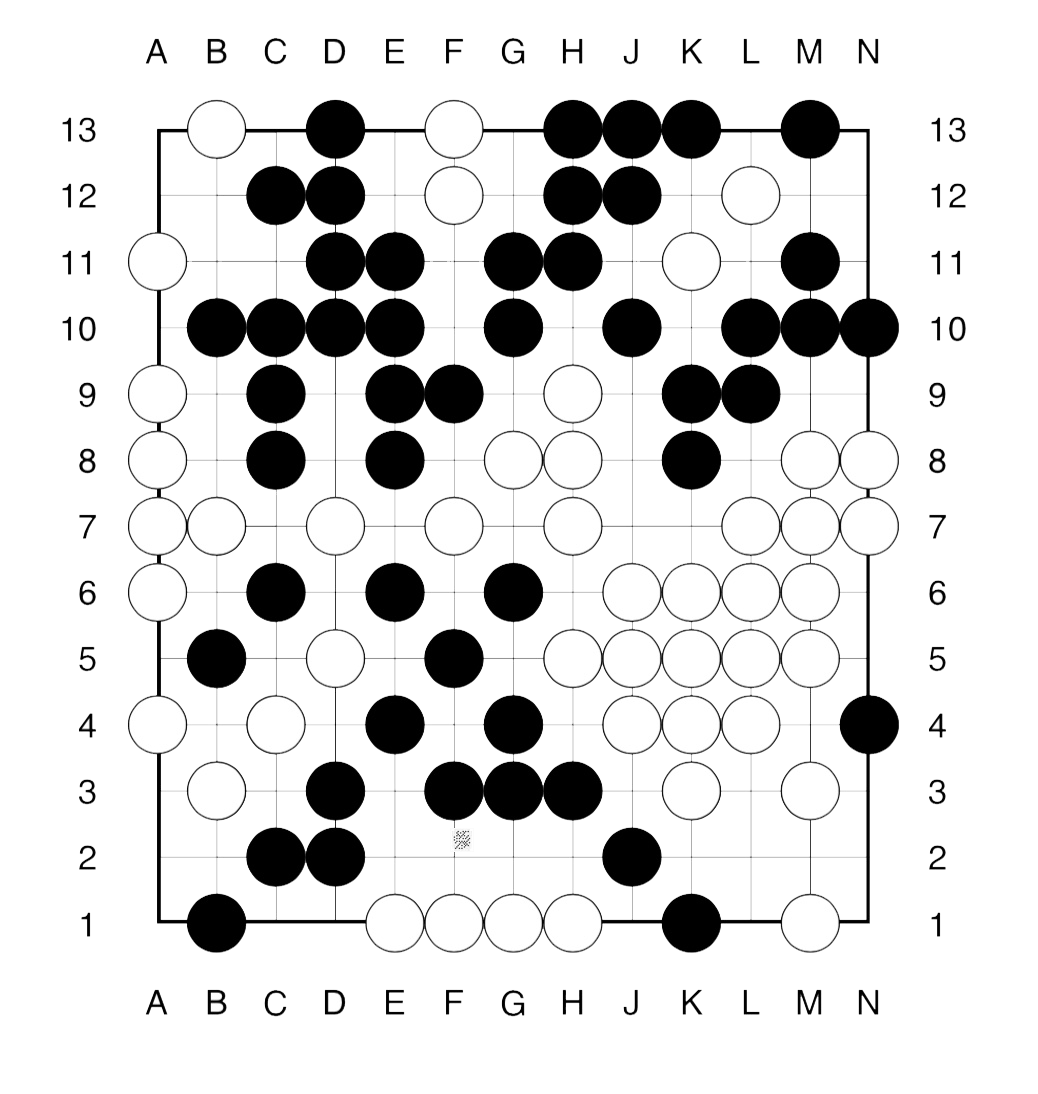The method to approach such an endgame is similar to that in COLgo. First we remove all dead spots from the board.

What remains, are a 4 spots available only to Right (white) and 5 spots available only to Left (bLack). Further, there a 3 ‘live’ regions: the upper righthand corner and the two lower corners.

The value of these corners must be computed inductively.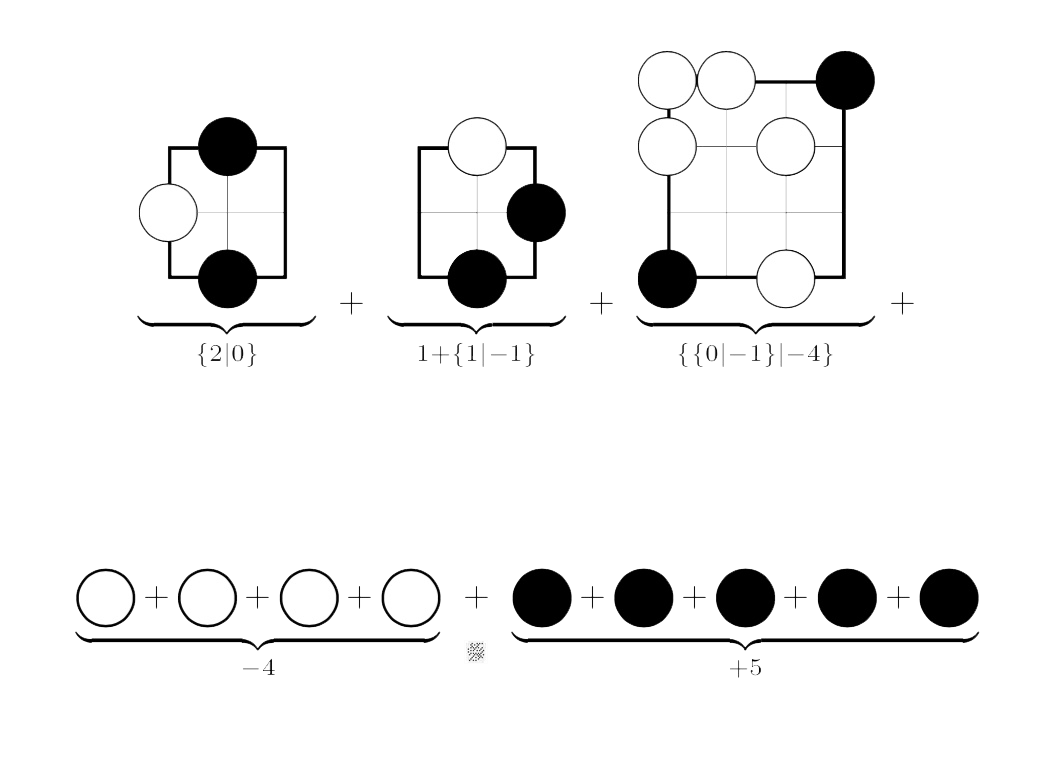For example, Right’s best option in the left-most game (corresponding to the upper righthand corner of the endgame) is to put his stone on N12, resulting in a game in which neither player can move (the zero game).

On the other hand, Left can put a stone at either N11, N12 or N13 leaving a game in which she has two more moves, whereas Right han none (the $2$ game).

The other positions are computed similarly.

To get the value of the endgame we have to sum up all these values.

This can either be done using the addition rule given in ONAG, or by using programs in combinatorial game theory.

There’s Combinatorial Game Suite, developed by Aaron Siegel. But, for some reason I can no longer use it on macOS High Sierra.

Fortunately, the older program David Wolfe’s toolkit is still available, and runs on my MacBook.

The sum game evaluates to $\{ \{3|2 \}|-1 \}$, which is a ‘fuzzy’ game, that is, its value is confused with $0$.

This means that the first player to move has a winning strategy in the endgame.

Can you spot the (unique) winning move for Right (white) and one (of two) winning move for Left (bLack)?